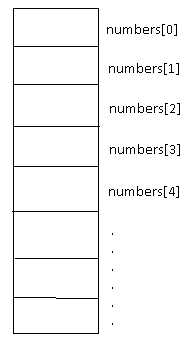# Arrays

An array is a linear data structure. The array elements are represented in the memory as sequential memory locations. Arrays are easy to traverse, search and sort. They are used to store relatively permanent collections of data. It consists of numbered list of items where each item is of same type.

An array is of fixed length. Let’s create an array of 10 integers.

Int[] numbers = new int;

Arrays are placed in contiguous memory locations.

### Representation of array in memory

The arrays are represented in memory as contiguous memory location as shown below:The array members are accessed by index number for example, number represents first member of array numbers. In most of programming languages, the index of array starts from 0.

Searching is one of the most extensive operations performed in an array. Let’s discuss two such searching algorithms:

• Linear search
• Binary search

## Linear Search

The simplest searching method in an array is linear search. It works on any kind of array i.e. sorted or unsorted one. The steps involved are:

1. The searching starts at beginning of array.
2. Then it matches each element one by one until the end of array is reached.

This algorithm is considered as in worst case the whole array needs to be traversed. The average case complexity of linear search is n/2.

## Binary Search

One of the effective searching methodologies is binary search. The binary search works on sorted array. The steps in binary search are:

1. It looks for the element in the middle of array.
2. If element is not found, then it ignores the half of array and repeat the process on other half of the array.

In every step, the number of elements to search is reduced by half. The complexity of binary search algorithm is log2n.